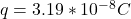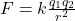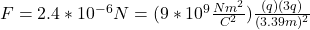## Kalyan ramji sain, of india, had a mustache that measured 3.39 m from end to end in 1993. suppose two charges, q and 3q, are placed 3.39 m a

Question

Kalyan ramji sain, of india, had a mustache that measured 3.39 m from end to end in 1993. suppose two charges, q and 3q, are placed 3.39 m apart. if the magnitude of the electric force between the charges is 2.4 × 10−6 n, what is the value of q?

in progress 0
5 months 2021-08-02T21:48:29+00:00 1 Answers 35 views 0

## Answers ( )Explanation:

The expression for the electric force between two chargeswhere K is the Coulomb’s constant (k=9*10^{9}Nm^2/C^2), and we have that

q1=q

q2=3q

r=3.39m

F=2.4*10^{-6}N

By replacing in the formula we haveand by taking apart q we havehope this helps!!